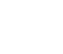## Physics P3 Questions and Answers - Nambale Mock Exams 2021/2022

Instructions to candidates

• Write your name, index number, school and date in the spaces provided above
• Sign and write the date of the examination in the spaces provided above
• Answer all the questions in the spaces provided
• All writings must be shown in the spaces provided

FOR EXAMINER’S USE ONLY

 Question 1 b (i) b (ii) d e (i) e (ii) e (iii) TOTAL Maximum score 1 1 7 5 3 3 20 Candidate score
 Question 1 c e (i) e (ii) e (iii) h (i) h (ii) TOTAL Maximum score 1 7 5 2 2 3 20 Candidate score## Question

Question One
You are provided with the following

• Two cells of 1.5V each
• Nichrome wire labeled W mounted on a metre rule
• An ammeter (0 – 1.5A) or (0 - 2.5A)
• A cell holder
• Voltmeter (0 – 5V)
• 8 connecting wires atleast 4 with crocodile clips (or a jockey)
• A switch
• A metre rule

Proceed as follows:

1. Connect the circuit as shown in the figure 1 below.Figure 1
2.
1. Connect the end of A and point B when AB = 100cm across the terminals as shown in the figure 1 above.
Close the switch and measure both current I and p.d, V across the wire AB
Current I....................... A
P.d, V........................ V
2. Measure the emf of the cell, E = V
3. Reduce the length AB as shown, 100cm, 70cm, 60cm, 50cm, 40cm, 30cm and 20cm. In each case record the current (I) and the corresponding values of p.d (V)
4. Enter the length as shown in the table 1 below:
 Length AB (cm) 100 70 60 50 40 30 20 Current I (A) p.d (V) E – V (V)
Table 1
5. Complete the table
1. Plot a graph of (E – V) against I (A)
2. Determine the gradients of the graphGiven the equation, E = V =Ir, determine the internal resistance of each cell (3mks)

Question Two
Part A

You are provided with the following apparatus

• A wooden metre rule
• Masses, two of 10g and two of 20g
• Knife edge – 20cm high

Proceed as follows

1. Arrange the apparatus as shown in figure 2 below
2. Balance the metre rule on the edge and adjust the metre rule until it balances horizontally when there is no mass on it. The knife edge is now at the position of (cog)
3. Record the position of (cog)
Position of c.o.g = ..................................cm
Figure 24. Now hang a mass on the metre rule by use of the thread at 1cm mark. Adjust the knife edge until the metre rule balances again at new mark. Record the length d1 and the corresponding length d2.
1. Repeat the procedure for different masses and complete the table 2 shown below.
Table 2
 Mass, m(g) 10 20 30 40 50 60 Distance d1 (cm) Distance d2 (cm) Mass (m) x distance d2 =md2
2. Plot a graph of md2 against d1 (5mks)3. Calculate the slopes of the graph (2mks)

Part B
You are provided with the following apparatus

• A lens
• A lens holder
• A candle
• A white screen
• A metre rule

Procedure
e). Set up the apparatus as shown in the figure 3 below:f). Starting with u = 30cm adjust the position of the screen to obtain a sharp image of the candle. Record value of V in the table shown below:
g).

1. Repeat the procedure above for u = 20cm and complete table below:
Table 3
 u (cm) v (cm) M = v/u 20 30
(2mks)
2. Given that the focal length of the lens satisfies the equation,determine the average value of the focal length## MARKING SCHEME

Question One

1.
1. I = 0.5 ± 0.05A  1⁄2 mk
p.d = 2.50 ± 0.2V  1⁄2 mk
2.  e.m.f, E = 3.0V ± 0.2 1 mk
2.
 Length AB (cm) 100 70 60 50 40 30 20 Current (A) 0.5 0.6 0.65 0.7 0.75 0.8 0.9 ± 0.05 p.d (V) 2.5 2.3 2.25 2.15 2.1 2 1.9 ± 0.2 E – V (V) 0.5 0.7 0.75 0.85 0.9 1 1.1
3.
1.  Graph
• Axis labeled with units
• Scale: uniform and simple
• 4 correctly plotted points
• Line through at least 3 correctly plotted points
Total 5mks
=∆Y
∆X
=∆(E-V)
∆I
=0.5
0.3
=5=1.67 V
3          A
Internal substitution
Correct evaluation
Accuracy
Total 3mks
NB: No line no slopeQuestion Two
Part A
(c) c.o.g = 50.0 ± 0.5 1
(e) (i)

 Mass, m (g) 10 20 30 40 50 60 Distance, d1 (cm) 3.7 7 10 12.3 14.4 16.4 Distance, d2 (cm) 44.8 41.5 38.5 36.2 34.2 32.2 Mass, (cm) xDistance, d2 (md2) 448 830 1155 1448 1710 1932 V1• Axis labeled with units (1mk)
• Scale simple and uniform (1mk)
• Atleast 4 correctly plotted points (2mks)
• Line through atleast 3 correctly plotted points (1mk)
Total marks 5mks

=∆Y
∆X
=(∆md2)
(∆d1)
=(360-444)
(12.4-4)
=(-84)
8.4
=-10

• 1 Evaluation
Total 2mks
 u (cm) v (cm) m =v/u 20 60 3 30 30 1

f=v/(1+m)=60/(1+3)=10cm+2cm
f=v/(1+m)=30/(1+1)=15cm+2cm
Average Value=(15+15)/2
=15cm±2cm## CONFIDENTIAL

Question One

• Two dry cells of 1.5V each.
• Nichrome wire labeled W mounted on a metre rule.
• An ammeter (0-1.5A) or (0-2.5A)
• A cell holder.
• Voltmeter.
• 8 connecting wires atleast 4 wash crocodile clips (or a Jockey)
• A switch.
• A metre rule.

Question Two

• A metre rule
• Two masses of 10g.
• Two masses of 20g.
• Knife edge, 20cm high.
• A candle.
• A lens holder.
• A white screen.
• Converging len of focal length 15cm.

• ✔ To read offline at any time.
• ✔ To Print at your convenience
• ✔ Share Easily with Friends / Students